# CLASS 9 MATHS CHAPTER-15 PROBABILITY

### Exercise 15.1

Question 1. In a cricket match a batsman hits a boundary 6 times out of 30 balls he plays. Find the probability that he will

(i) hit a boundary in the next ball.

(ii) not hit boundary in the next ball.

Solution:
For all solutions, Probability =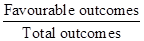(i) Number of times on boundary is hit = 6

∵ P (hit a boundary) =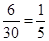(ii) Number of times on boundary is not hit = 30 – 6 = 24

∴  P (did not hit a boundary) =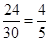Question 2. 1500 families with 2 children were selected randomly, and the following data were recorded:

Compute the probability of a family, chosen at random, having

(i) 2 girls (ii) 1 girl (iii) No girl

Also check whether the sum of these probabilities is 1.

Solution:

(i) Total number of families = 1500

No. of families having 2 girls = 475

∴ P (Family having 2 girls) =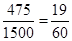(ii) No of families having 1 girl = 814

∴  P (Family having 1 girl) =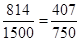(iii) No. of families having no girl = 211

∴ P (Family having no girl) = 211/1500

Checking: Sum of all probabilities =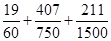= 1

Yes, the sum of probabilities is 1.

Question 3. In a particular section of Class IX, 40 students were asked about the months of their birth and the following graph was prepared for the data so obtained:

Find the probability that a student of the class was born in August.

Solution:

From the bar graph, we observe,

Total no. of students of Class IX = 40

No. of students of Class IX born in August = 6

∴ P (A student born in August) =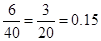Question 4. Three coins are tossed simultaneously 200 times with the following frequencies of different outcomes:

If the three coins are simultaneously tossed again, compute the probability of 2 heads coming up.

Solution:
No. of 2 heads = 72

Total number of outcomes = 23 + 72 + 77 + 28 = 200

∴ P (2 heads) =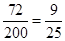Question 5. An organization selected 2400 families at random and surveyed them to determine a relationship between income level and the number of vehicles in a family. The information gathered is listed in the table below:

Suppose a family is chosen. Find the probability that the family chosen is:

(i) earning Rs. 10000 – 13000 per month and owning exactly 2 vehicles.

(ii) earning Rs. 16000 or more per month and owning exactly 1 vehicle.

(iii) earning less than Rs. 7000 per month and does not own any vehicle.

(iv) earning Rs. 13000 – 16000 per month and owning more than 2 vehicles.

(v) not more than 1 vehicle.

Solution:
(i) P (earning Rs. 10000 – 13000 per month and owning exactly 2 vehicles) =  29/2400

(ii) P (earning Rs. 16000 or more per month and owning exactly 1 vehicles) = 579/2400

(iii) P (earning Rs. 7000 per month and does not own any vehicles) =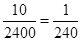(iv) P (earning Rs. 13000 – 16000 per month and owning more than 2 vehicles) =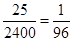(v) P (owning not more than 1 vehicle) =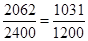Question 6. A teacher wanted to analyse the performance of two sections of students in a mathematics test of 100 marks. Looking at their performances, she found that a few students got under 20 marks and a few got 70 marks or above. So she decided to group them into intervals of varying sizes as follows: 0 − 20, 20 − 30… 60 − 70, 70 − 100. Then she formed the following table:

(i) Find the probability that a student obtained less than 20 % in the mathematics test.

(ii) Find the probability that a student obtained marks 60 or above.

Solution:
(i) No. of students obtaining marks less than 20 out of 100, i.e. 20% = 7

Total students in the class = 90

∴ P (A student obtained less than 20%) =  7 /90

(ii) No. of students obtaining marks 60 or above = 15 + 8 = 23

∴ P (A student obtained marks 60 or above) = 23 /90

Question 7. To know the opinion of the students about the subject statistics, a survey of 200 students was conducted. The data is recorded in the following table.

Find the probability that a student chosen at random

(i) likes statistics, (ii) does not like it

Solution:
Total no. of students on which the survey about the subject of statistics was conducted = 200

(i) No. of students who like statistics = 135

∴ P (a student likes statistics) =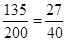(ii) No. of students who do not like statistics = 65

∴ P (a student does not like statistics) =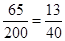Question 8. Refer Q.2, Exercise 14.2. What is the empirical probability than an engineer lives:

(i) less than 7 km from her place of work?

(ii) more than or equal to 7 km from her place of work?

(iii) within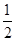km from her place of work?

Solution:
Total number of engineers = 40

(i) No. of engineers living less than 7 km from her place of work = 9

∴ P (Engineer living less than 7 km from her place of work) = 9 / 40

(ii) No. of engineers livingmore than or equal to 7 km from her place of work = 21

∴ P (Engineer living more than or equal to 7 km from her place of work) =

(iii) No. of engineers living withinkm from her place of work = 0

∴ P (Engineer living withinkm from her place of work) = 0/40= 0

Question 9. Activity: Note the frequency of two wheelers, three wheelers and four wheelers going past during a time interval, in front of your school gate. Find the probability that any one vehicle out of the total vehicles you have observed is a two wheeler.

Solution:
Let you noted the frequency of types of wheelers after school time (i.e. 3 pm to 3.30 pm) for half an hour.

Let the following table shows the frequency of wheelers.

Probability that a two wheelers passes after this interval =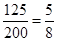Question 10. Ask all the students in your class to write a 3-digit number. Choose any student from the room at random. What is the probability that the number written by her/him is divisible by 3? Remember that a number is divisible by 3, if the sum of its digits is divisible by 3.

Solution:

This is an activity based question. Students are advised to perform this activity by yourself.

Question 11. Eleven bags of wheat flour, each marked 5 kg, actually contained the following weights of four (in kg): 4.97, 5.05, 5.08, 5.03, 5.00, 5.06, 5.08, 4.98, 5.04, 5.07, 5.00
Find the probability that any of these bags chosen at random contains more than 5 kg of flour.

Solution:
Number of bags containing more than 5 kg of wheat flour = 7

Total number of wheat flour bags = 11

∴ P (a bag containing more than 5 kg of wheat flour) = 7 /11

Question 12.

The above frequency distribution table represents the concentration of sulphur dioxide in the air in parts per million of a certain city for 30 days. Using this table, find the probability of the concentration of sulphur dioxide in the interval 0.12 − 0.16 on any of these days.

Solution:
From the frequency distribution table we observe that:

No. of days during which the concentration of Sulphur dioxide lies in interval 0.12 – 0.16 = 2

Total no. of days during which concentration of Sulphur dioxide recorded = 30

∴ P (day when concentration of Sulphur dioxide (in ppm) lies in 0.12 – 0.16) =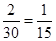Question 13.

The above frequency distribution table represents the blood groups of 30 students of a class. Use this table to determine the probability that a student of this class, selected at random, has blood group AB.

Solution:
From the frequency distribution table we observe that:

Number of students having blood group AB = 3

Total number of students whose blood group were recorded = 30

∴ P (a student having blood group AB) =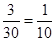Get 30% off your first purchase!

X
error: Content is protected !!
Scroll to Top